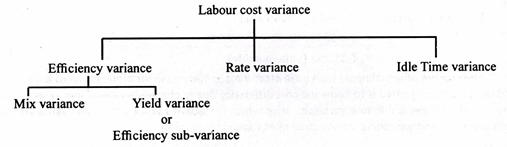# Labour Variance

08/04/2020

Direct labour variances arise when actual labour costs are different from standard labour costs. In analysis of labour costs, the emphasis is on labour rates and labour hours.

Labour variances constitute the following:

#### Labour Cost Variance:Labour cost variance denotes the difference between the actual direct wages paid and the standard direct wages specified for the output achieved.

This variance is calculated by using the following formula:

Labour cost variance = (AH x AR) – (SH x SR)

Where:

AH = Actual hours

AR = Actual rate

SH = Standard hours

SR = Standard rate

Labour Efficiency Variance:

The calculation of labour efficiency or usage variance follows the same pattern as the computa­tion of materials usage variance. Labour efficiency variance occurs when labour operations are more efficient or less efficient than standard performance. If actual direct labour hours required to complete a job differ from the number of standard hours specified, a labour efficiency variance results; it is the difference between actual hours expended and standard labour hours specified multiplied by the stand­ard labour rate per hour.

Labour efficiency variance is computed by applying the following formula:

Labour efficiency variance = (Actual hours – Standard hours for the actual output) x Std. rate per hour.

Assume the following data:

Standard labour hour per unit = 5 hr

Standard labour rate per hour = Rs 30

Units completed = 1,000

Labour cost recorded = 5,050 hrs @ Rs 35

Labour efficiency variance = (5,050-5,000) x Rs 30 = Rs 1,500 (unfavourable) It may be noted that the standard labour hour rate and not the actual rate is used in computing labour efficiency variance. If quantity variances are calculated, changes in prices/rates are excluded, and when price variances are calculated, standard quantities are ignored.

(i) Labour Mix Variance:

Labour mix variance is computed in the same manner as materials mix variance. Manufacturing or completing a job requires different types or grades of workers and production will be complete if labour is mixed according to standard proportion. Standard labour mix may not be adhered to under some circumstances and substitution will have to be made. There may be changes in the wage rates of some workers; there may be a need to use more skilled or expensive types of labour, e.g., employ­ment of men instead of women; sometimes workers and operators may be absent.

These lead to the emergence of a labour mix variance which is calculated by using the following formula:

Labour mix variance = (Actual labour mix – Revised standard labour mix in terms of actual total hours) x Standard rate per hour

(ii) Labour Yield Variance:

The final product cost contains not only material cost but also labour cost. Therefore, gain or loss (higher or lower output than the standard output) should take into account labour yield variance also. A lower output simply means that final output does not correspond with the production units that should have been produced from the hours expended on the inputs.

It can be computed by ap­plying the following formula:

Labour yield variance = (Actual output – Standard output based on actual hours) x Av. Std. Labour Rate per unit of output.

Or

Labour yield variance = (Actual loss – Standard loss on actual hours) x Average standard labour rate per unit of output

Labour yield variance is also known as labour efficiency sub-variance which is computed in terms of inputs, i.e., standard labour hours and revised labour hours mix (in terms of actual hours).

Labour efficiency sub-variance is computed by using the following formula:

Labour efficiency sub-variance = (Revised standard mix – standard mix) x Standard rate

Labour Rate Variance:

Labour rate variance is computed in the same manner as materials price variance. When actual direct labour hour rates differ from standard rates, the result is a labour rate variance. It is that portion of the direct wages variance which is due to the difference between actual rate paid and standard rate of pay specified.

The formula for its calculation is:

Labour rate variance = (Actual rate – Standard rate) x Actual hours

Using data from the example given above, the labour rate variance is Rs 25,250, i.e.,

Labour rate variance = (35 – 30) x 5050 hours = 5 x 5050 = Rs 25,250 (unfavourable)

The number of actual hours worked is used in place of the number of the standard hours speci­fied because the objective is to know the cost difference due to change in labour hour rates, and not hours worked. Favourable rate variances arise whenever actual rates are less than standard rates; unfavourable variances occur when actual rates exceed standard rates.

Idle Time Variance:

Idle time variance occurs when workers are not able to do the work due to some reason during the hours for which they are paid. Idle time can be divided according to causes responsible for creat­ing idle time, e.g., idle time due to breakdown, lack of materials or power failures. Idle time variance will be equivalent to the standard labour cost of the hours during which no work has been done but for which workers have been paid for unproductive time.